GFG App
Open AppBrowser
Continue

# Newton’s Third Law of Motion

You’re undoubtedly aware that throwing a ball against a wall causes the ball to exert force on the wall. Similarly, the wall exerts a force on the ball, causing it to bounce off the wall. Similarly, the earth’s gravitational attraction pushes you down. What you may not understand is that you are also putting equal power on the ground. This amazing statistic is a result of Newton’s third law.

Newton’s Third Law states that if object A exerts a force on another item B, object B must exert a force of equal size and opposite direction on object A. This rule represents a specific symmetry in nature: forces always exist in pairs, and one body cannot exert a force on another without also suffering a force.

Let us explore Newton’s third law in-depth as,

What are Newton’s Laws of Motion?

The branch of physics dealing with the simulated motions of all large-scale and familiar bodies in the universe, for instance, the cars, planets, and humans come under classical mechanics, stipulated by Newton. In comparison, the motion of minutely small atomic and sub-atomic objects is covered under the branch of quantum mechanics, stipulated by Euler.

The following are Newton’s Laws of Motion :

1. Law of Inertia- Newton’s first law states that, if a body is at rest or moving at a constant speed in a straight line, it will remain at rest or keep moving in a straight line at constant speed unless it is acted upon by an external acting force.
2. The second law states that the rate of change of momentum of an object is directly proportional to the force applied for any object of constant mass.
3. The third law states that when one object exerts a force on a second object, that second object exerts a force that is equal in magnitude and opposite in direction on the first object.

### Newton’s Third Law of Motion

Newton’s Third Law of Motion states that: “To every action there is always an equal and opposite reaction.” Action and reaction forces are applied on different bodies at the same instance time frame. The system maintains its equilibrium.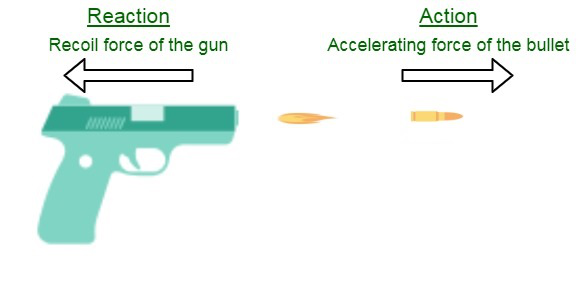According to Newton’s third law, when two bodies engage, they apply forces to each other that are equal in magnitude and opposing in direction. The third law is also known as the action-reaction law. This law is useful in assessing static equilibrium issues in which all forces are balanced, but it also applies to things in constant or accelerated motion.

The forces it explains are genuine, not just techniques. A book, for example, sitting on a table exerts a downward force equal to its weight on the table. The table exerts an equal and opposite force on the book, according to the third law. The weight of the book causes the table to slightly bend, causing it to press back on the book like a coiled spring.

Mathematically, Newton’s Third Law of Motion can also be written as shown below: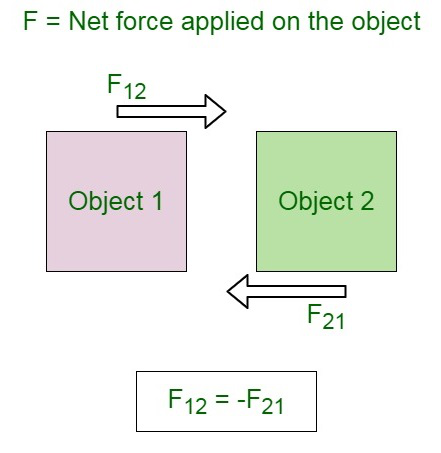Here, F12 is the force applied by body 1 on body 2 and F21 is the force applied by body 2 on body 1. The minus sign indicates that the two forces are applied in a mutually opposite direction.

Forces always occur in pairs. If two bodies A and B exist within a system, then the force of A on B and force of B and A become internal forces of the system, and they cancel each other since they are opposite in direction and equal. As a result, the system maintains its equilibrium.

### Newton’s Third Law of Motion in Nature

• The propulsion of a fish through the water – A fish uses its fins to push water backward to simulate its motion. In turn, the water exerts an equal reacting force by pushing the fish forward, propelling the fish through the water.
• The magnitude of the force exerted by the fish on the water is equivalent to the size of the force on the fish.
• The direction of the force on the water which is backward in nature is opposite the direction of the forward force on the fish.
• The fly of birds through the air –  The flying motion of birds is governed by the birds pushing down on the air with their wings, while the air in return pushes their wings up and gives them lift.
• The direction of the force on the air which is backward in nature is opposite the direction of the forward force on the birds.
• Action-reaction force pairs make it possible for birds to fly.

### Proof that Action and Reaction are Equal and Opposite

To understand the concept of the action and reaction forces, let us consider a system of two spring balances A and B connected together.

The spring balance B is fixed to any rigid support. A force is then applied in the loose and free end, by pulling the spring balance A. As an effect of the application of force, both the spring balances show the same readings.

This concludes that both the spring balances witness equal magnitude forces. It also shows that the force exerted by both the spring balances, A on B is equal but opposite in direction to the force exerted by spring balance B on A.

Here, he force exerted on the acting body (the spring balance A on B) is termed as action, and the force exerted by the reacting body (spring balance B on A) can be termed as reaction.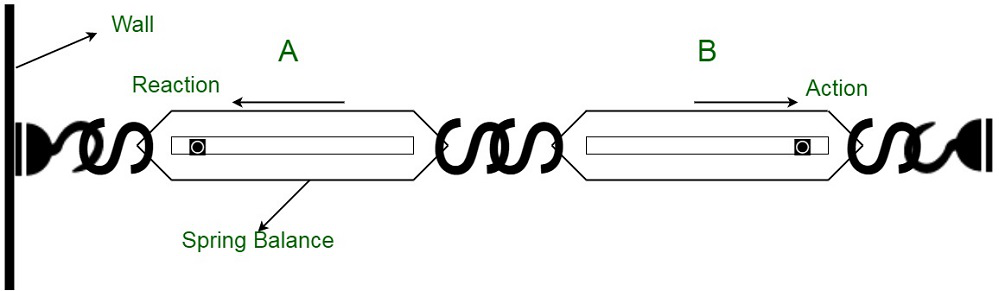### Application of Newton’s Third Law of Motion

• Bullet Reconcile: When a bullet is fired from a gun, it exerts a forward force (action) on the bullet and the bullet exerts an equal and opposite force on the gun (reaction), and the gun recoils. The reaction force is experienced on the hand of the firing person.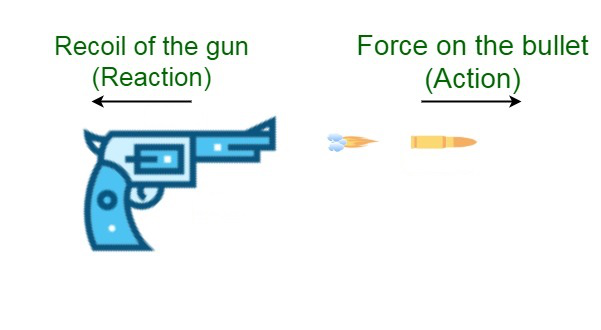• When a sailor jumps out of a boat, he pushed the boat backward by exerting a backward force of the boat (action) and he jumps forward. Thereby, the boat exerts an equal and opposite force on the sailor called reaction.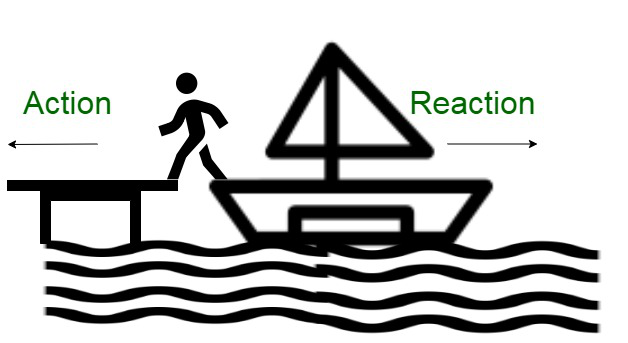• When we release an air-filled balloon, the force of the air (gases) coming out of the balloon is the action, which exerts an equal and opposite force on the balloon called reaction by moving upward.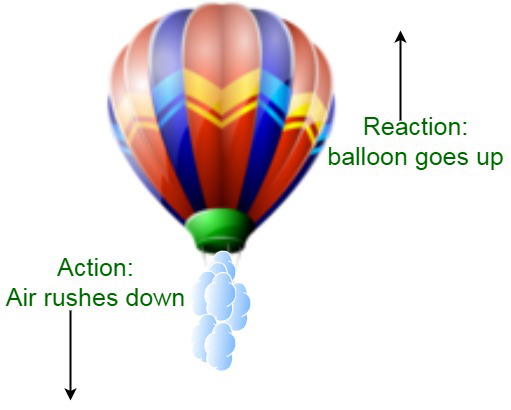• When a rocket is fired, the force of the burning gases coming out (action) exerts an equal and opposite force on the rocket (reaction) and it moves upward.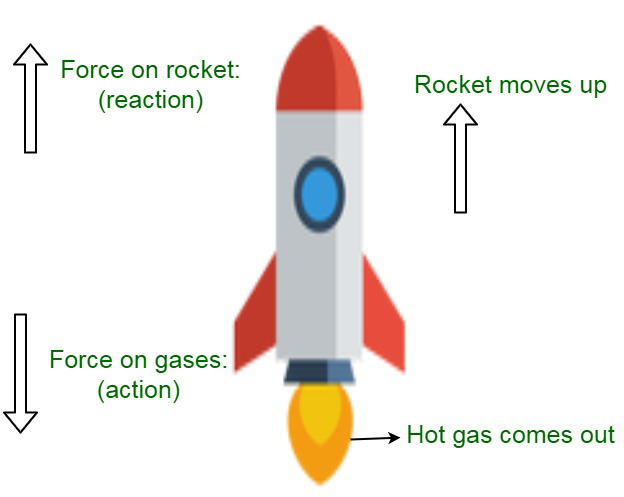Different kinds of fuels are burned in the rocket’s engine, producing hot gases. These gases push against the inside tube of the rocket. After igniting and burning through the inside tube, the gases escape from the bottom of the rocket.  As these gases flow downward, the rocket rises in the sky towards the upward direction. Therefore, as the gases and rocket move in the opposite direction w.r.t each other. The reaction of a rocket is the application of the third law of motion.

### Mathematical Interpretation of Newtons Third Law of Motion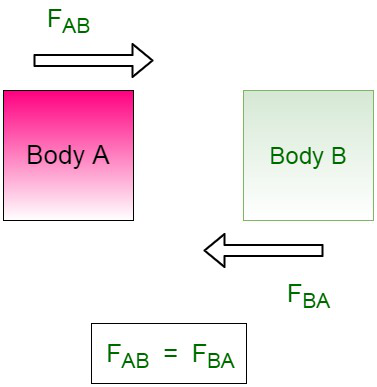Newton’s third law of motion states that every action has equal and opposite reaction.

For a system of two bodies, A and B, let us assume FAB is a force of body A acting on B and FBA is force by B  on body A.

The mathematical expression w.r.t the forces is given by,

FAB = – FBA

where, FAB is an action on B while FBA is reaction of body B on A.

The negative sign indicates that force acting on body A is in opposite direction to the force which is acting on body B.

### Derivation of Newton’s Third Law of Motion from Newton’s Second Law of Motion

Let us assume an isolated system with no external forces acting upon them, consisting of two massive bodies A & B mutually interacting with each other. Now, Let us consider both bodies to be in effect of a force under the influence of each other, that is, FAB, to be the force exerted on body B by body A and FBA be the force exerted by body B on A.

Due to these forces, FAB and FBA, let us assume dp1/dt and dp2/dt to be the rate of the change of momentum in the effect of these bodies respectively.Then,

FBA = dp1/dt and FAB = dp2/dt

FBA + FAB = dp1/dt + dp2/dt

= d(p1 + p2) / dt

Since, no external force acts on the system, therefore:

d(p1 + p2) / dt = 0

or

FBA + FAB = 0

FBA = -FAB

The above equation represents Newton’s third law of motion (i.e, for every action there, is an equal and opposite reaction).

### Sample Problems

Problem 1: A car with a mass of 1250 Kg traveling by an acceleration of 10 m/s2 hits a bike. What force does the car experience?

Solution:

According to Newton’ second law,

The force on the bike, F = m × a

= 1250  kg × 10 m/s2

= 12500 N

Now according to Newton’s third law, for every action, there is an equal and opposite reaction.

Thus, the car experiences a force of 10 N.

Problem 2: A Dog of mass 10 kg jumps on a table of mass 60 kg. As the Dog walks around on the table, what is the average force that the table applies to the Dog? Use g = 10 m/s2.

Solution:

The force that the dog applies to the table is its weight. As per Newton’s third law, the table also applies a force to the dog of the same magnitude.

The force on the dog from the table is:

Fs = FN = ma = 10 kg × 10 m/s2 = 100 N

Problem 3: A boy is riding his scooter and pushes off the ground with his foot. Thus this causes him to accelerate at a rate of 8 m/s2. Boy’s weight is 600 N. What is the strength of his push off the ground? Use g = 10 m/s2.

Solution:

Boy’s weight, F is 600 N.

The formula to calculate the force on an object is,

F = ma

where m is the mass and a is the acceleration.

or

m = F / a

= 600 N / 10 m/s2

= 60 kg

Boy accelerates at 8 m/s2. so, he is pushed by a force of

F = ma = 60 kg × 8 m/s2 = 480 N

Problem 4: Two bodies apply forces to each other. The force on one of the bodies as a function of time in the x-direction is kt + b, where k and b are constants. What’s the force as a function of time in the x-direction on the other body? Consider no other forces are present besides the forces the bodies apply to each other.

Solution:

According to Newton’s third law, Every action has an equal and opposite reaction.

Thus, the force has an equal and opposite force on the other body.

Mathematically, this just means to negative the force. Therefore, the force as a function of time in the x-direction on the other body is -ky – b.

Problem 5: What happens to the magnitudes of force and acceleration of the gun and the bullet, when a player shoots a gun and the force of the shot results in recoil?

Solution:

According to Newton’s third law, every force has an equal and opposite reaction, the force on the gun is equal to the force on the bullet.

Therefore, the gun has a larger mass, so the magnitude of its acceleration is less than that of the bullet. A player shoots a gun and the force of the shot results in recoil. The magnitude of the force on the gun equals the magnitude of the force on the bullet, and the magnitude of the acceleration of the gun is less than that of the bullet.

My Personal Notes arrow_drop_up